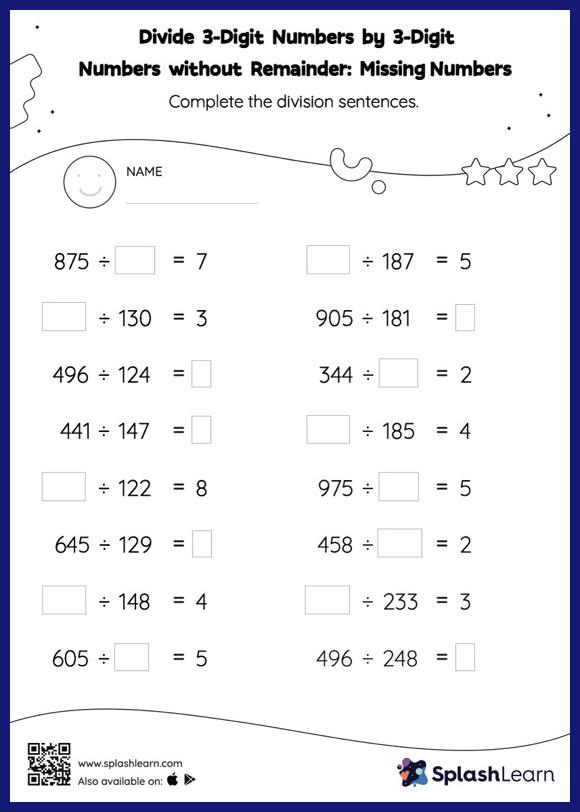# Divide 3-Digit Numbers by 3-Digit Numbers without Remainder: Missing Numbers Worksheet

Home > Divide 3-Digit Numbers by 3-Digit Numbers without Remainder: Missing NumbersDivide 3-digit numbers by 3-digit numbers without remainder worksheet helps students develop proficiency with division. In divide 3-digit numbers by 3-digit numbers without remainder worksheet, students find the missing number using their understanding of division facts and the long division method. No remainders exist for this set of problems.